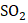# A mixture ofandin the molar ratiois diffused through a pin hole for successive effusions three times to give a molar ratioof diffused mixture. Which of the following are not correct if diffusion is made at sameandin each operation? a) Eight operations are needed to getmolar ratio b) Rate of diffusion forafter 8 operations isc) Six operations are needed to getmolar ratio forandin diffusion mixture d) Rate of diffusion forandafter 6 operations is## Question ID - 102746 :- A mixture ofandin the molar ratiois diffused through a pin hole for successive effusions three times to give a molar ratioof diffused mixture. Which of the following are not correct if diffusion is made at sameandin each operation? a) Eight operations are needed to getmolar ratio b) Rate of diffusion forafter 8 operations isc) Six operations are needed to getmolar ratio forandin diffusion mixture d) Rate of diffusion forandafter 6 operations is3537

 Eight operations are needed to getmolar ratio Rate of diffusion forafter 8 operations isSix operations are needed to getmolar ratio forandin diffusion mixture
 Which of the following statements is/are true? a) Hydrogen diffuses four times faster than oxygen b) The temperature of a real gas changes when it expands adiabatically in vacuum c) An ideal gas undergoes cooling effect when it suffers an adiabatic expansion in vacuum d) The Joule-Thomson coefficientof an ideal gas is zero